# 图文详解 DFS 和 BFS

danielmou

0收藏

### 前言

• 深度优先遍历，广度优先遍历简介
• 习题演练
• DFS，BFS 在搜索引擎中的应用

### 深度优先遍历，广度优先遍历简介

#### 深度优先遍历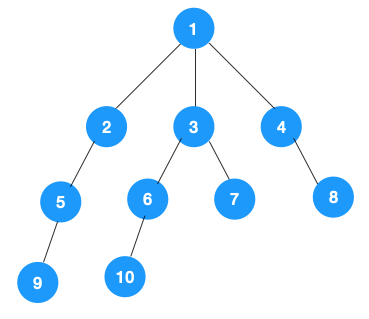1、我们从根节点 1 开始遍历，它相邻的节点有 2，3，4，先遍历节点 2，再遍历 2 的子节点 5，然后再遍历 5 的子节点 9。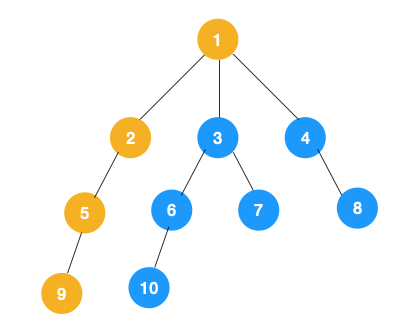2、上图中一条路已经走到底了（9是叶子节点，再无可遍历的节点），此时就从 9 回退到上一个节点 5，看下节点 5 是否还有除 9 以外的节点，没有继续回退到 2，2 也没有除 5 以外的节点，回退到 1，1 有除 2 以外的节点 3，所以从节点 3 开始进行深度优先遍历，如下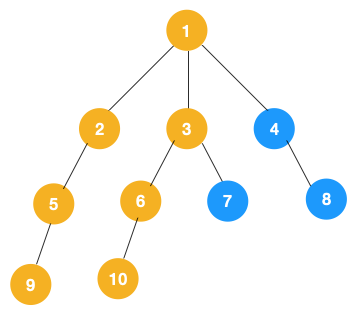3、同理从 10 开始往上回溯到 6, 6 没有除 10 以外的子节点，再往上回溯，发现 3 有除 6 以外的子点 7，所以此时会遍历 7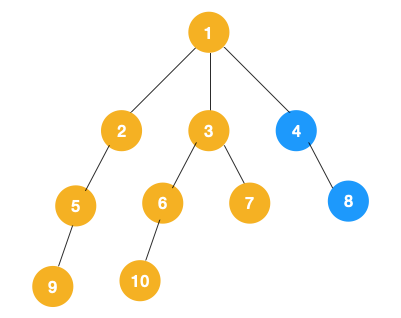3、从 7 往上回溯到 3， 1，发现 1 还有节点 4 未遍历，所以此时沿着 4， 8 进行遍历,这样就遍历完成了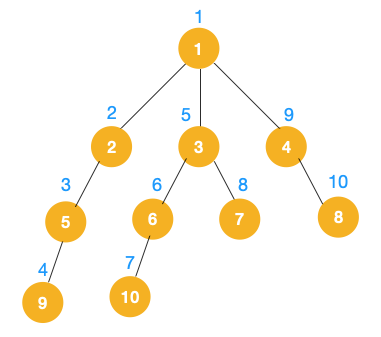1、递归实现

``````    public     class Solution {
private     static     class Node {
/**
* 节点值
*/
public     int value;
/**
* 左节点
*/
public Node left;
/**
* 右节点
*/
public Node right;

public Node(    int{
this.value = value;
this.left = left;
this.right = right;
}
}

public     static     void dfs(Node treeNode){
if (treeNode ==     null) {
return;
}
// 遍历节点
process(treeNode)
// 遍历左节点
dfs(treeNode.left);
// 遍历右节点
dfs(treeNode.right);
}
}``````

2、非递归实现

1. 对于每个节点来说，先遍历当前节点，然后把右节点压栈，再压左节点（这样弹栈的时候会先拿到左节点遍历，符合深度优先遍历要求）
2. 弹栈，拿到栈顶的节点，如果节点不为空，重复步骤 1， 如果为空，结束遍历。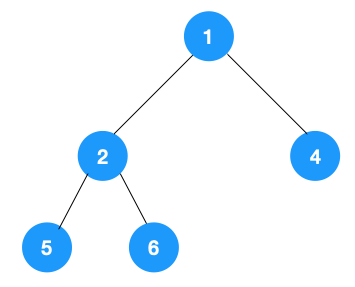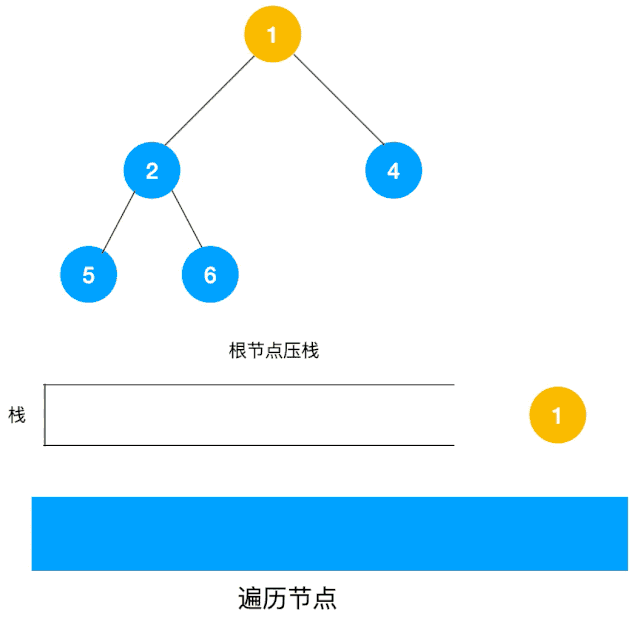``````/**
* 使用栈来实现 dfs
* @param root
*/
public     static     void dfsWithStack(Node root){
if (root ==     null) {
return;
}

Stack<Node> stack =     new Stack<>();
// 先把根节点压栈
stack.push(root);
while (!stack.isEmpty()) {
Node treeNode = stack.pop();
// 遍历节点
process(treeNode)

// 先压右节点
if (treeNode.right !=     null) {
stack.push(treeNode.right);
}

// 再压左节点
if (treeNode.left !=     null) {
stack.push(treeNode.left);
}
}
}``````

#### 广度优先遍历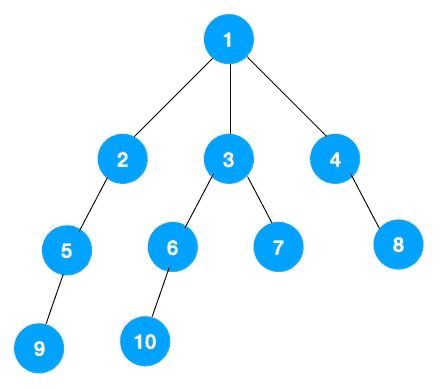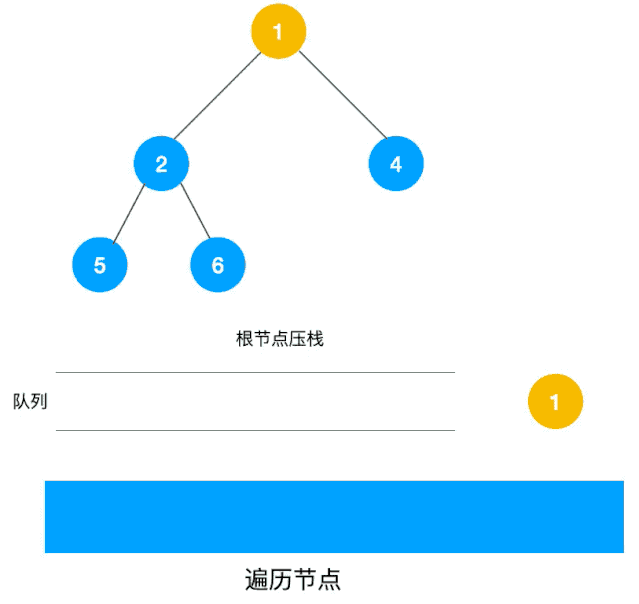``````/**
* 使用队列实现 bfs
* @param root
*/
private     static     void bfs(Node root){
if (root ==     null) {
return;
}

while (!stack.isEmpty()) {
Node node = stack.poll();
System.out.println("value = " + node.value);
Node left = node.left;
if (left !=     null) {
}
Node right = node.right;
if (right !=     null) {
}
}
}``````

### 习题演练

leetcode 104，111: 给定一个二叉树，找出其最大/最小深度。

``````    3
/ \
9  20
/  \
15   7``````

``````/**
* leetcode 104: 求树的最大深度
* @param node
* @return
*/
public     static     int getMaxDepth(Node node){
if (node ==     null) {
return 0;
}
int leftDepth = getMaxDepth(node.left) + 1;
int rightDepth = getMaxDepth(node.right) + 1;
return Math.max(leftDepth, rightDepth);
}

/**
* leetcode 111: 求树的最小深度
* @param node
* @return
*/
public     static     int getMinDepth(Node node){
if (node ==     null) {
return 0;
}
int leftDepth = getMinDepth(node.left) + 1;
int rightDepth = getMinDepth(node.right) + 1;
return Math.min(leftDepth, rightDepth);
}``````

leetcode 102: 给你一个二叉树，请你返回其按层序遍历得到的节点值。（即逐层地，从左到右访问所有节点）。示例，给定二叉树：[3,9,20,null,null,15,7]

``````    3
/ \
9  20
/  \
15   7``````

``````[
,
[9,20],
[15,7]
]``````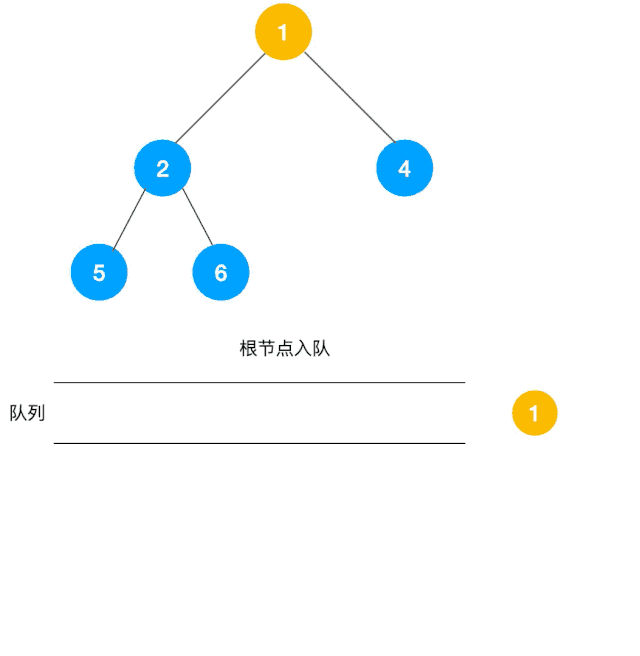Java 代码

``````/**
* leetcdoe 102: 二叉树的层序遍历, 使用 bfs
* @param root
*/
private     static List<List<Integer>> bfsWithBinaryTreeLevelOrderTraversal(Node root) {
if (root ==     null) {
// 根节点为空，说明二叉树不存在，直接返回空数组
return Arrays.asList();
}

// 最终的层序遍历结果
List<List<Integer>> result =     new ArrayList<>();

queue.offer(root);

while (!queue.isEmpty()) {
// 记录每一层
List<Integer> level =     new ArrayList<>();
int levelNum = queue.size();
// 遍历当前层的节点
for (    int i = 0; i < levelNum; i++) {
Node node = queue.poll();
// 队首节点的左右子节点入队,由于 levelNum 是在入队前算的，所以入队的左右节点并不会在当前层被遍历到
if (node.left !=     null) {
}
if (node.right !=     null) {
}
}
}

return result;
}``````

Python 代码:

``````    class Solution:
def levelOrder(self, root):
"""
:type root: TreeNode
:rtype: List[List[int]]
"""
res = []  #嵌套列表，保存最终结果
if root     is None:
return res

from collections     import deque
que = deque([root])  #队列，保存待处理的节点
while len(que)!=0:
lev = []  #列表，保存该层的节点的值
thislevel = len(que)  #该层节点个数
while thislevel!=0:
#队首节点的左右孩子入队
thislevel-=1
res.append(lev)
return res``````

``````    private     static     final List<List<Integer>> TRAVERSAL_LIST  =     new ArrayList<>();
/**
* leetcdoe 102: 二叉树的层序遍历, 使用 dfs
* @param root
* @return
*/
private     static     void dfs(Node root,     int{
if (root ==     null) {
return;
}

if (TRAVERSAL_LIST.size() < level + 1) {
}

List<Integer> levelList = TRAVERSAL_LIST.get(level);

// 遍历左结点
dfs(root.left, level + 1);

// 遍历右结点
dfs(root.right, level + 1);
}``````

### DFS，BFS 在搜索引擎中的应用

1、网页抓取

2、预处理

3、排名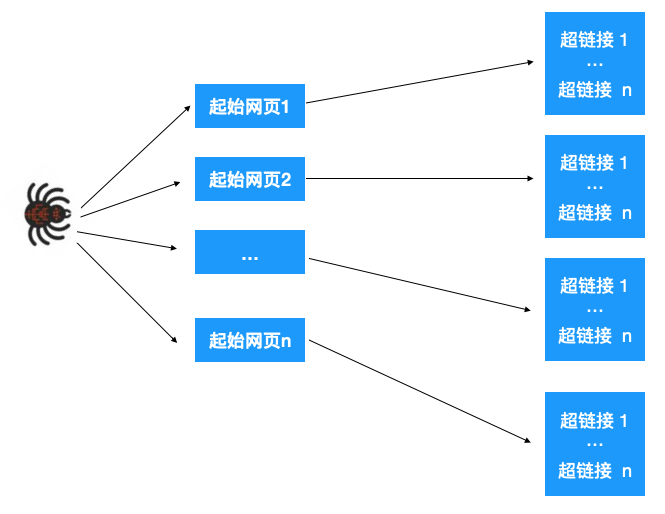### 总结

DFS 和 BFS 是非常重要的两种算法，大家一定要掌握，本文为了方便讲解，只对树做了 DFS，BFS，大家可以试试如果用图的话该怎么写代码，原理其实也是一样，只不过图和树两者的表示形式不同而已，DFS 一般是解决连通性问题，而 BFS 一般是解决最短路径问题，之后有机会我们会一起来学习下并查集，Dijkstra, Prism 算法等，敬请期待！